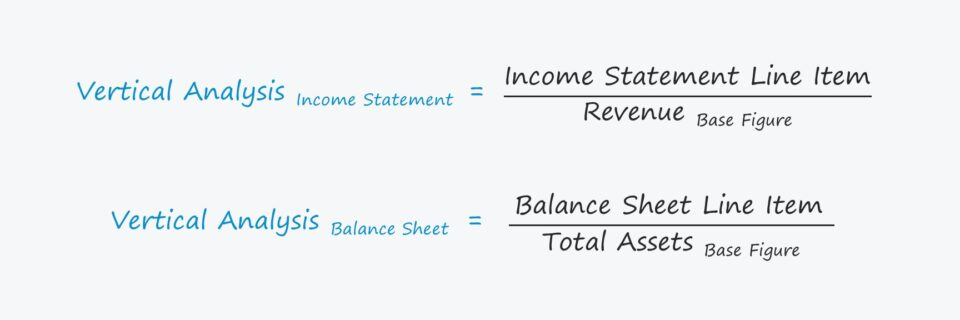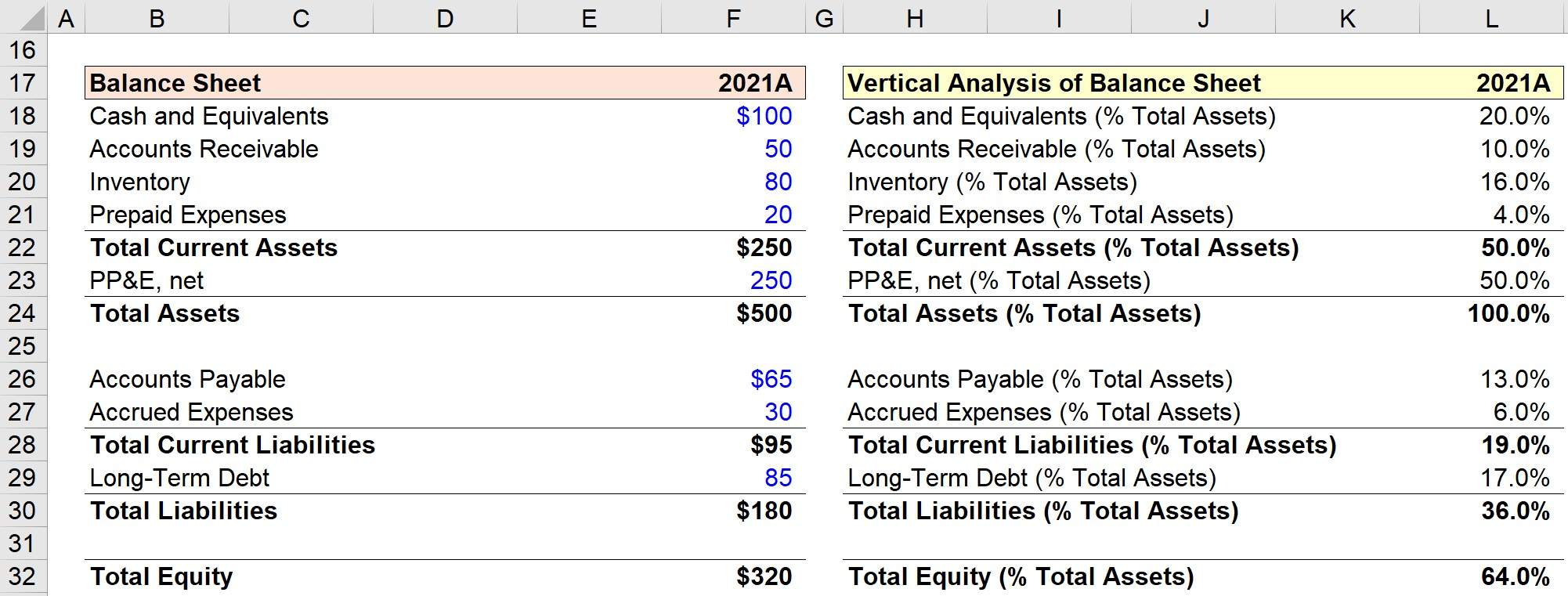Welcome to Wall Street Prep! Use code at checkout for 15% off.# Vertical Analysis

Guide to Understanding Vertical Analysis## How to Perform Vertical Analysis?

Conceptually, vertical analysis can be thought of as reading a single column of financial data and determining the relationships among each item to reflect the relative size of the various cost and profit metrics.

The standard base figures for the income statement and balance sheet are as follows.

• Income Statement → The base figure for the income statement is most often revenue, or sales (i.e. the “top line”), so each expense and profitability metric is expressed as a percentage of revenue. A less common base metric for the income statement, yet still informative, is the total operating expenses line item, which can be used to assess the percentage breakdown of a company’s operating expenses (e.g. research and development, selling, general and administrative)
• Balance Sheet → On the other hand, the base figure for the balance sheet is usually the “Total Assets” line item for all sections, although “Total Liabilities” can also be used. Note that by dividing a company’s liabilities and equity line items by total assets, you’re essentially dividing by the sum of those two sections because of the accounting equation (i.e. Assets = Liabilities + Shareholders Equity).

## Common Size Analysis of Financial Statements

Performing vertical analysis creates the so-called “common size” income statement and the “common size” balance sheet.

The common size financials are denoted in percentage terms, which facilitates direct comparisons between the target company and its peer group of comparable companies, such as competitors operating in the same or an adjacent industry (i.e. an “apples-to-apples” comparison).

Unlike the unadjusted income statement and balance sheet, the common size variations can be used for peer-to-peer comparisons between different companies.

## Vertical Analysis Formula

Starting from the revenue line item, each line item on the income statement – if deemed appropriate – is divided by revenue (or the applicable core metric).

The formula to perform vertical analysis on the income statement, assuming the base figure is revenue, is as follows.

Vertical Analysis, Income Statement = Income Statement Line Item ÷ Revenue

In contrast, the process is practically the same for the balance sheet, but there is the added option of using “Total Liabilities” instead of “Total Assets”. But we’ll utilize the latter here, as that tends to be the more prevalent approach taken.

Vertical Analysis, Balance Sheet = Balance Sheet Line Item ÷ Total Assets

## Vertical Analysis Calculator

We’ll now move to a modeling exercise, which you can access by filling out the form below.Submitting...

## Step 1. Historical Income Statement and Balance Sheet Data

Suppose we’ve been tasked with performing vertical analysis on a company’s financial performance in its latest fiscal year, 2021.

To start, the table below shows the company’s historical financial statements – the income statement and balance sheet – of our hypothetical company, which we’ll be using throughout our two-part exercise.

Historical Income Statement 2021A
Revenue \$200 million
Less: COGS (120) million
Gross Profit \$80 million
Less: SG&A (25) million
Less: R&D (10) million
EBIT \$45 million
Less: Interest Expense (5) million
EBT \$40 million
Less: Taxes (30%) (12) million
Net Income \$28 million
Historical Balance Sheet 2021A
Cash and Equivalents \$100 million
Accounts Receivable 50 million
Inventory 80 million
Prepaid Expenses 20 million
Total Current Assets \$250 million
PP&E, net 250 million
Total Assets \$500 million
Accounts Payable \$65 million
Accrued Expenses 30 million
Total Current Liabilities \$95 million
Long-Term Debt 85 million
Total Liabilities \$180 million
Total Equity \$320 million

Once the historical data from 2021 has been inputted into Excel, we must determine the base figure to use.

Here, we’ve chosen “Revenue” as the base figure for the common size income statement, followed by “Total Assets” for the common size balance sheet.

## Step 2. Vertical Analysis of Income Statement

### Percentage of Revenue Calculation

With our financial data presented in Excel, we can start to calculate the contribution percentages on either the side or below the income statement.

Regardless of the placement, the more important factor is to ensure the analysis clearly shows which period it is reflecting.

The placement is not much of a concern in our simple exercise, however, the analysis can become rather “crowded” given numerous periods.

So if we had multiple years of historical data, it is recommended to organize the percentage calculations into a single section on the far right or below the financials with the timing of the periods aligned.

In order to keep a complex model more dynamic and intuitive to the reader(s), it is generally a “best practice” to avoid creating separate columns in between each period.

Further, when working with large data sets, we recommend cleaning up the data to improve the overall visual representation of the analysis.

For example, some minor adjustments could be to remove the “Revenue (% Revenue)” line item since it is not necessary and offers no practical insights.

For each line item, we’ll divide the amount by the corresponding period’s revenue to arrive at our contribution percentages.

Because we entered our costs and expenses as negatives, i.e. to reflect that those items are cash outflows, we must place a negative sign in front when applicable, so that the percentage shown is a positive figure.

Of the takeaways from our common size income statement, the most important metrics are the following:

Vertical Analysis of Income Statement 2021A
Revenue (% Revenue) 100.0%
COGS (% Revenue) (60.0%)
Gross Margin (%) 40.0%
SG&A (% Revenue) (12.5%)
R&D (% Revenue) (5.0%)
Operating Margin (%) 22.5%
Interest Expense (% Revenue) (2.5%)
EBT Margin (%) 20.0%
Taxes (% Revenue) (6.0%)
Net Profit Margin (%) 14.0%

## Step 3. Vertical Analysis of Balance Sheet

### Percentage of Total Assets Calculation

We’ve now completed our vertical analysis for our company’s income statement and will move on to the balance sheet.

The process is virtually identical to our common size income statement, however, the base figure is “Total Assets” as opposed to “Revenue”.

Once we divide each balance sheet item by the “Total Assets” of \$500 million, we are left with the following table.

The assets section is informative with regard to understanding which assets belonging to the company constitute the greatest percentage.

In our case, half of the company’s asset base comprises PP&E, with the rest coming from its current assets.

The sum of the current assets equals 50%, confirming our calculations thus far are correct.

On the liabilities and shareholders equity side, we’ve chosen the base figure to be total assets.

To reiterate from earlier, dividing by total assets is akin to dividing by the sum of liabilities and equity.

Since liabilities and equity represent a company’s funding sources – i.e. how the company obtained the funds to purchase its assets – this part of the analysis can be insightful for understanding where the company’s financing stems from.

For instance, we can see that our company’s long-term debt as a percentage of total assets is 17.0%. The metric we calculated is formally known as the “debt to asset ratio”, which is a ratio used to gauge a company’s solvency risk and the proportion of its resources (i.e. assets) funded by debt rather than equity.

Vertical Analysis of Balance Sheet 2021A
Cash and Equivalents (% Total Assets) 20.0%
Accounts Receivable (% Total Assets) 10.0%
Inventory (% Total Assets) 16.0%
Prepaid Expenses (% Total Assets) 4.0%
Total Current Assets (% Total Assets) 50.0%
PP&E, net (% Total Assets) 50.0%
Total Assets (% Total Assets) 100.0%
Accounts Payable (% Total Assets) 13.0%
Accrued Expenses (% Total Assets) 6.0%
Total Current Liabilities (% Total Assets) 19.0%
Long-Term Debt (% Total Assets) 17.0%
Total Liabilities (% Total Assets) 36.0%
Total Equity (% Total Assets) 64.0%Step-by-Step Online Course

### Everything You Need To Master Financial Modeling

Enroll in The Premium Package: Learn Financial Statement Modeling, DCF, M&A, LBO and Comps. The same training program used at top investment banks.